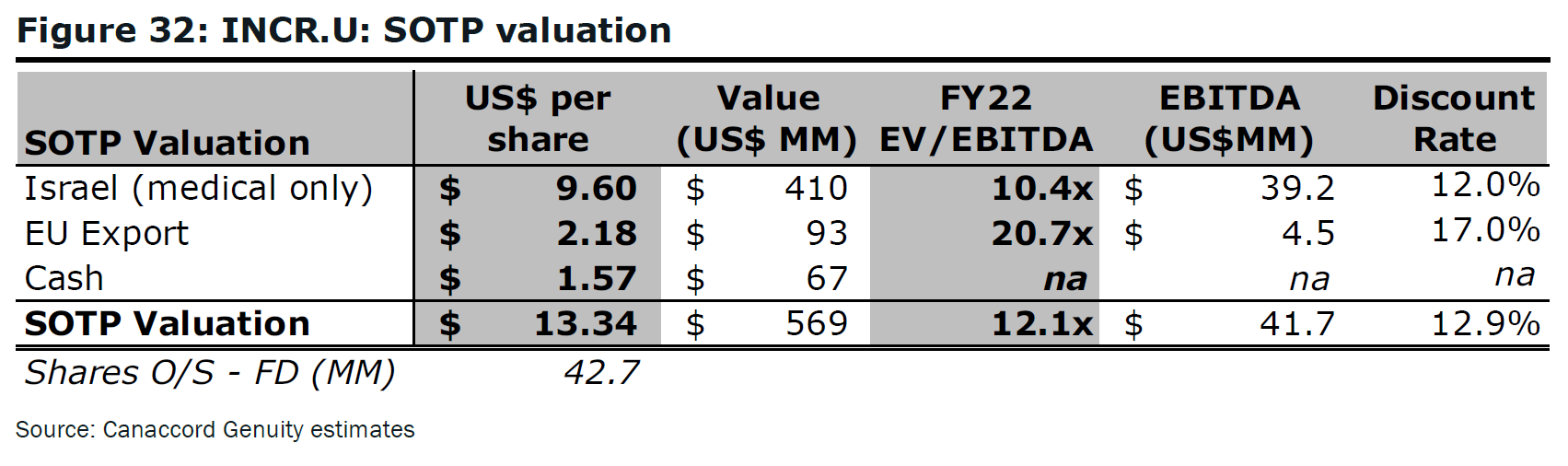# Fight Finance

#### CoursesTagsRandomAllRecentScores

One year ago a pharmaceutical firm floated by selling its 1 million shares for $100 each. Its book and market values of equity were both$100m. Its debt totalled $50m. The required return on the firm's assets was 15%, equity 20% and debt 5% pa. In the year since then, the firm: • Earned net income of$29m.
• Paid dividends totaling $10m. • Discovered a valuable new drug that will lead to a massive 1,000 times increase in the firm's net income in 10 years after the research is commercialised. News of the discovery was publicly announced. The firm's systematic risk remains unchanged. Which of the following statements is NOT correct? All statements are about current figures, not figures one year ago. Hint: Book return on assets (ROA) and book return on equity (ROE) are ratios that accountants like to use to measure a business's past performance. $$\text{ROA}= \dfrac{\text{Net income}}{\text{Book value of assets}}$$ $$\text{ROE}= \dfrac{\text{Net income}}{\text{Book value of equity}}$$ The required return on assets $r_V$ is a return that financiers like to use to estimate a business's future required performance which compensates them for the firm's assets' risks. If the business were to achieve realised historical returns equal to its required returns, then investment into the business's assets would have been a zero-NPV decision, which is neither good nor bad but fair. $$r_\text{V, 0 to 1}= \dfrac{\text{Cash flow from assets}_\text{1}}{\text{Market value of assets}_\text{0}} = \dfrac{CFFA_\text{1}}{V_\text{0}}$$ Similarly for equity and debt. Which of the following statements about book and market equity is NOT correct? If a firm makes a profit and pays no dividends, which of the firm’s accounts will increase? Question 768 accounting terminology, book and market values, no explanation Accountants and finance professionals have lots of names for the same things which can be quite confusing. Which of the following groups of items are NOT synonyms? Here is a table from Canaccord's 'sum of the parts' valuation of INCR.Note that the firm INCR is unlevered (interest bearing debt = 0). The third column from the left is labelled 'Value (US$ MM)'. For the 'Israel' and 'EU Export' opportunities, these values are most likely to be: